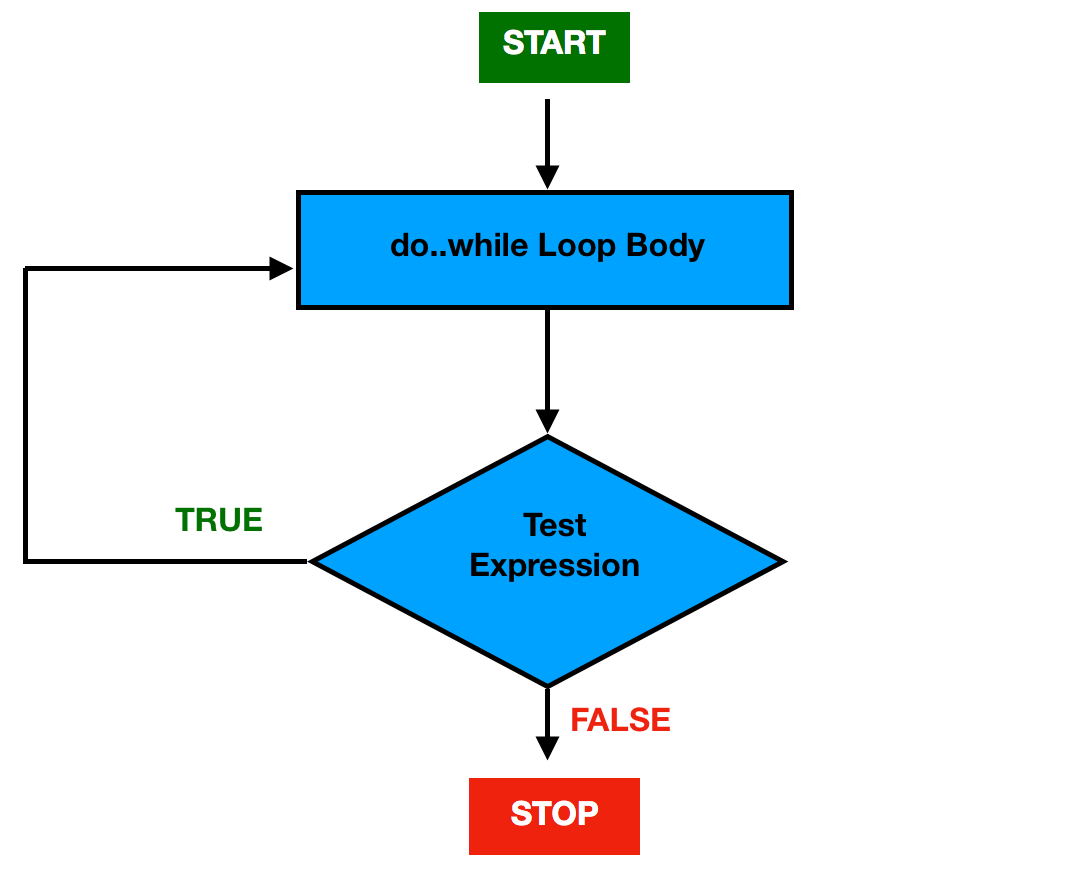# Java do-while Loop

In this article, you will learn about the do-while loop and how to create do-while loop in Java programming.

Loops in Java are used when we want to execute a block of statements repeatedly until a specific condition is met(condition is false).

### Java do-while Loop

Java do-while loop is an exit-control loop, which means unlike while loop, a do-while loop check for the condition after executing the statements or the loop body.

The do-while loop is similar to while loop with one main difference. The body of the do-while loop is executed at least once before the test-expression is checked.

Note: the do-while loop will execute the statements at least once before any condition is checked.

#### Syntax

```do {
//body of the loop
//statements to be executed
}
//test expression is a boolean expression
while (test_expression);```

### Flowchart of do-while loop### How do-while loop works?

When the Control falls into the do-while loop, it first executes the body of the loop inside the do block and then it checks the test_expression.

If the test expression is evaluated to true,

• The statements inside the do block(body of the loop) get executed.
• Then, the test expression is evaluated again and this process goes on until the test expression is evaluated to false.

If the test expression is evaluated to false,

• do-while loop gets terminated and control goes out of the do-while loop.

#### Example 1

```public class DoWhileLoopDemo {

public static void main(String[] args) {

int i = 1;

do {
System.out.println("value of i: " + i);

//increment value of i by 1
i++;
}
//exit when i becomes greater than 3
while (i < 4);
}
}```

#### Output

```value of i: 1
value of i: 2
value of i: 3```

#### Example explained

In the above example,

1. i is initialized with value 1.

2. Execute the loop body inside do block.

“value of i: 1” gets printed.

• Updation is done. Now i = 2.

3. Condition 2 < 4 is checked, yields true.

4. Execute the loop body inside do block.

• “value of i: 2” gets printed.
• Updation is done. Now i = 3.

5. Condition 3< 4 is checked, yields true.

6. Execute the loop body inside do block.

• “value of i: 3” gets printed.
• Updation is done. Now i = 4.

7. Condition 4< 4 is checked, yields false.

8. Exit from the do-while loop.

#### Example 2

The below program will print the sum of natural numbers from 1 to 10.

```public class DoWhileLoopDemo {

public static void main(String[] args) {

int i = 1, sum = 0;

do {

sum = sum + i;

//Increment the value of i for
//next iteration
i++;
}

// Exit when i becomes greater than 10
while (i <= 10);

System.out.println("Sum of numbers from 1 to 10: " + sum);
}
}```

#### Output

`Sum of numbers from 1 to 10: 55`